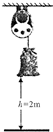（1）重物下落到地面的过程中，重力做的总功为360J；

（2）重物下落到地面的过程中，重力做功的功率为0.2W；

（3）该装置把重力做的功转化为电能的效率为75%。

#### 解析：

(1) 重力做的总功: $W=G h=m g h=18 \mathrm{~kg} \times 10 \mathrm{~N} / \mathrm{kg} \times 2 \mathrm{~m}=360 \mathrm{~J}$;
(2) 重物下落过程中重力做功的功率:
$\mathrm{P}_{\text {重力 }}=\frac{\mathbb{W}}{\mathrm{t}}=\frac{360 \mathrm{~J}}{0.5 \times 3600 \mathrm{~s}}=0.2 \mathrm{~W} ;$
(3) 3 只灯泡正常发光时的总功率: $P$ 电 $=3 \times 0.05 \mathrm{~W}=0.15 \mathrm{~W}$, 该装置把重力做的功转化为电能的效率:
①点击 收藏 此题， 扫码注册关注公众号接收信息推送（一月四份试卷,中1+高2+研1）
② 程序开发、服务器资源都需要大量的钱，如果你感觉本站好或者受到到帮助，欢迎赞助本站,赞助方式：微信/支付宝转账到 18155261033

 ①此题难易度如何 ②此题推荐度如何 确定